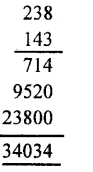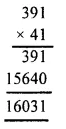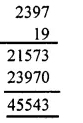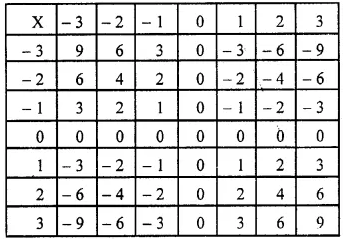## RS Aggarwal Class 6 Solutions Chapter 4 Integers Ex 4D

These Solutions are part of RS Aggarwal Solutions Class 6. Here we have given RS Aggarwal Solutions Class 6 Chapter 4 Integers Ex 4D.

Other Exercises

Question 1.
Solution:
(i) 15 by 9 = 15 x 9 = 135
(ii) 18 by – 7 = 18 x ( – 7) = – 126
(iii) 29 by – 11 = 29 x ( – 11) = – 319
(iv) – 18 by 13 = ( – 18) x 13 = – 234
(v) – 56 by 16 = ( – 56) x 16 = – 896
(vi) 32 by – 21 = 32 x ( – 21) = – 672
(vii) – 57 x 0 = ( – 57) x 0 = 0
(viii) 0 by – 31 = 0 x ( – 31) = 0
(ix) – 12 by – 9 = ( – 12) x ( – 9) = 108
(x) – 746 by – 8 = ( – 746) x ( – 8) = 5968
(xi) 118 by – 7 = 118 x ( – 7) = – 826
(xii) – 238 by – 143 = ( – 238) x ( – 143) = 238 x 143 = 34034Question 2.
Solution:
(i) ( – 2) x 3 x ( – 4) = [( – 2) x 3] x ( – 4) = ( – 6) x ( – 4) = 24
(ii) 2 x ( – 5) x ( – 6) = 2 x [( – 5) x ( – 6)] = 2 x 30 = 60
(iii) ( – 8) x 3 x 5 = ( – 8) x (3 x 5) = ( – 8) x 15 = – 120
(iv) 8 x 7 x ( – 10) = (8 x 7) x ( – 10) = 56 x ( – 10) = – 560
(v) ( – 3) x ( – 7) x ( – 6) = [( – 3) x ( – 7)] x ( – 6) = 21 x ( – 6) = – 126
(vi) ( – 8) x ( – 3) x ( – 9) = ( – 8) x [( – 3) x ( – 9)] = ( – 8) x 27 = – 216

Question 3.
Solution:
(i) 18 x ( – 27) x 30 = 18 x [( – 27) x 30] = 18 x ( – 810) = – 14580
(ii) ( – 8) x ( – 63) x 9 = [( – 8) x ( – 63)] x 9 = 504 x 9 = 4536
(iii) ( – 17) x ( – 23) x 41 = [( – 17) x ( – 23)] x 41 = 391 x 41 = 16031(iv) ( – 51) x ( – 47) x ( – 19) = [( – 51) x ( – 47)] x ( – 19) = 2397 x ( – 19) = – 45543Question 4.
Solution:
(i) We have :
18 x [9 + ( – 7)] = 18 x 2 = 36
18 x 9 + 18 x ( – 7)
= (18 x 9) + [18 x ( – 7)]
= 162 – 126
= 36
18 x [9 x ( – 7)] = 18 x 9 + 18 x ( – 7) is verified.
(ii) We have :
( – 13) x [( – 6) x ( – 19)]
= ( – 13) x ( – 25) = 325
( – 13) x ( – 6) + ( – 13) x – 9
= [( – 13) x ( – 6)] + [( – 13) x ( – 19)]
= 78 + 247 = 325
( – 13) x [( – 6) + ( – 19)]
= ( – 13) x ( – 6) + ( – 13) x ( – 19) is verified.

Question 5.
Solution:
The complete multiplication table is given belowQuestion 6.
Solution:
(i) True
(ii) False
(iii) True
(iv) True

Question 7.
Solution:
(i) ( – 9) x 6 + ( – 9) x 4
= ( – 9) x (6 + 4)
(By distributive law)
= ( – 9) x 10
= – 90
(ii) 8 x ( – 12) + 7 x ( – 12)
= ( 8 + 7) x ( – 12)
(By distributive law)
= 15 x ( – 12)
= – 180
(iii) 30 x ( – 22) + 30 x (14)
= 30 x [( – 22) + 14]
(By distributive law)
= 30 x ( – 8)
= – 240
(iv) ( – 15) x ( – 14) + ( – 15) x ( – 6)
= ( – 15) x [( – 14) + ( – 6)]
(By distributive law)
= ( – 15) x ( – 20)
= 300
(v) 43 x ( – 33) + 43 x ( – 17)
= 43 x [( – 33) + ( – 17)]
(By distributive law)
= 43 x ( – 50) = – 2150
(vi) ( – 36) x 72 + ( – 36) x 28
= ( – 36) x (72 + 28)
(By distributive law)
= ( – 36) x 100
= – 3600
(vii)( – 27) x ( – 16) + ( – 27) x ( – 14)
= ( – 27) x [( – 16) + ( – 14)]
(By distributive law)
= ( – 27) x ( – 30)
= 810

Hope given RS Aggarwal Solutions Class 6 Chapter 4 Integers Ex 4D are helpful to complete your math homework.

If you have any doubts, please comment below. Learn Insta try to provide online math tutoring for you.